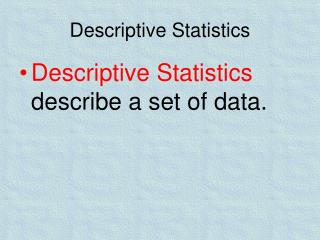DownloadDownload PresentationDescriptive Statistics

# Descriptive Statistics

Télécharger la présentation## Descriptive Statistics

- - - - - - - - - - - - - - - - - - - - - - - - - - - E N D - - - - - - - - - - - - - - - - - - - - - - - - - - -
##### Presentation Transcript

1. Descriptive Statistics • Descriptive Statistics describe a set of data.

2. If I want to report findings from an observation or a survey, I may want to use a frequency distribution. • A frequency distribution may be a simple chart, a list, or a graph. • A graph of information always plots the frequency along the y-axis, and the subject of the graph along the x-axis.

3. Measures of Central Tendency • Measures of central tendency provide statistics that indicate the average or typical score in the distribution. • Mean • Median • Mode • There are three measures of central tendency:

4. Mean • The mean is the arithmetic average of all the scores in the distribution. It is calculated by adding all the scores in the distribution and then dividing this sum by the number of scores.

5. Median The median is in the middle of the road! • The median is the middle score of the distribution, the point that divides a rank-ordered distribution into halves containing an equal number of scores. Thus 50% of the scores lie below the median and 50% lie above the median.

6. Mode • The mode is simply the score in the distribution that occurs most frequently.

7. Graphing Measures of Central Tendency • When graphing the mean, median and mode of a distribution, roughly speaking, a distribution has positive skewif the right tail is longer and negative skew if the left tail is longer.

8. Positively Skewed • This distribution has a positive skew. Note that the mean is larger than the median.

9. IE. In a neighborhood of relatively low incomes, a few millionaires move in. Those few high salaries will inflate the mean (average), but the median will remain relatively low.

10. Negatively Skewed • This distribution has a negative skew. The median is larger than the mean.

11. IE. In a particular well-to-do neighborhood, a few low-income residents move in. The overall average income will drop a bit, but the median will remain relatively high.

12. Measures of Variability • Measures of variability show how spread out the distribution of scores is from the mean, or how much dispersion or scatter exists in the distribution. If there is a large degree of dispersion, that is, if the scores are very dissimilar, we say the distribution has a large or high variability, or variance. If the scores are very similar, there is a small degree of dispersion and a small variance.

13. Measures of Variability • Range • Standard Deviation

14. Range • The range is simply the numerical difference between the highest and lowest scores in the distribution.

15. Standard Deviation • The measure of variability used most often in research is the standard deviation, a statistic that indicates the average distance of the scores from the mean of the distribution.

16. IE. Our class took Unit Exam 2. • I scored a 76%. • How did I do compared to everyone else in class? • I need to figure out what the class average was, figure out the standard deviation from the mean, and I’ll know how well I did.

17. Standard Deviation

18. With a mean of 62 and a SD of 3, 95% of scores should fall between what scores?

19. 15.Which of the following sets of numbers has the largest standard deviation? (a) –2, –1, 0, +1, +2 (b) 1.00, 1.25, 1.50, 1.75, 2.00, 2.25, 2.50, 2.75, 3.00 (c) 2, 6, 10, 14, 18 (d) 5.756, 5.765, 5.890, 5.895, 5.923 (e) 91, 92, 93, 94, 95

20. Graphing Standard Deviation • Find the mean of your distribution set. • Calculate the SD on your calculator. • The mean is set at “0”. • +1 and -1 are your SD above and below the mean. • IE. Your mean is 56 with a SD of 6. +1 would be 62, and -1 would be 50. • Calculate +- 2 and +- 3 in the same manner.

21. Graphing Standard Deviation • What does this tell us? • If the mean of a set of class scores on a unit exam was 72, with a SD of 8, 68% of students scored between a 64 and an 80. Your score of a 76 would be close to being better than 68% of the rest of the class. • Approximately 95% of the class scored between a 56 and an 88. Your score of a 50 would indicated that roughly 96% of the class did better than you on the test.

22. Graphing Data • Scatterplot – A graphed cluster of dots that represent the values of two variables.

23. The SLOPE of the points suggests whether there is a positive, negative, or non-existent relationship between two variables.

24. NEGATIVE CORRELATION – as one set of scores goes up, another set goes down

25. NO CORRELATION

26. No correlation relates to a score of 0.00 • A positive correlation ranges from 0.00 to 1.00 • A negative correlation ranges from 0.00 to -1.00

27. How closely the dots are to each other along the line indicates the strength or weakness of the correlation as well